How to Calculate and Solve for Young’s Modulus | Rock Mechanics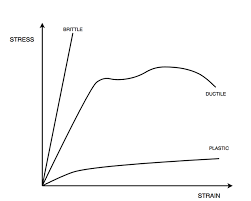The image above represents young’s modulus.

To compute for young’s modulus, two essential parameters are needed and these parameters are axial stress (σa) and axial strain (εa).

The formula for calculating the young’s modulus:

E = σa / εa

Where:

E = Young’s Modulus
σa = Axial Stress
εa = Axial Strain

Let’s solve an example;
Given that the axial stress is 65 and the axial strain is 45. Calculate for the young’s modulus?

This implies that;

σa = Axial Stress = 65
εa = Axial Strain = 45

E = σa / εa
E = 65 / 45
E = 1.44

Therefore, the young’s modulus is 1.44.

Calculating the Axial Stress when the Young’s Modulus and the Axial Strain is Given.

σa = E x εa

Where;

σa = Axial Stress
E = Young’s Modulus
εa = Axial Strain

Let’s solve an example;
Given that the young’s modulus is 25 and the axial strain is 10. Calculate for the axial stress?

This implies that;

E = Young’s Modulus = 25
εa = Axial Strain = 10

σa = E x εa
σa = 25 x 10
σa = 250

Therefore, the axial stress is 250.

Calculating the Axial Strain when the Young’s Modulus and the Axial Stress is Given.

εa = σa / E

Where;

εa = Axial Strain
E = Young’s Modulus
σa = Axial Stress

Let’s solve an example;
Given that the young’s modulus is 7 and the axial stress is 35. Calculate for the axial strain?

This implies that;

E = Young’s Modulus = 7
σa = Axial Stress = 35

εa = σa / E
εa = 35 / 7
εa = 5

Therefore, the axial strain is 5.

Nickzom Calculator – The Calculator Encyclopedia is capable of calculating the young’s modulus.

To get the answer and workings of the young’s modulus using the Nickzom Calculator – The Calculator Encyclopedia. First, you need to obtain the app.

You can get this app via any of these means:

To get access to the professional version via web, you need to register and subscribe for NGN 1,500 per annum to have utter access to all functionalities.
You can also try the demo version via https://www.nickzom.org/calculator

Android (Paid) – https://play.google.com/store/apps/details?id=org.nickzom.nickzomcalculator
Android (Free) – https://play.google.com/store/apps/details?id=com.nickzom.nickzomcalculator
Apple (Paid) – https://itunes.apple.com/us/app/nickzom-calculator/id1331162702?mt=8
Once, you have obtained the calculator encyclopedia app, proceed to the Calculator Map, then click on Geology under Add-on.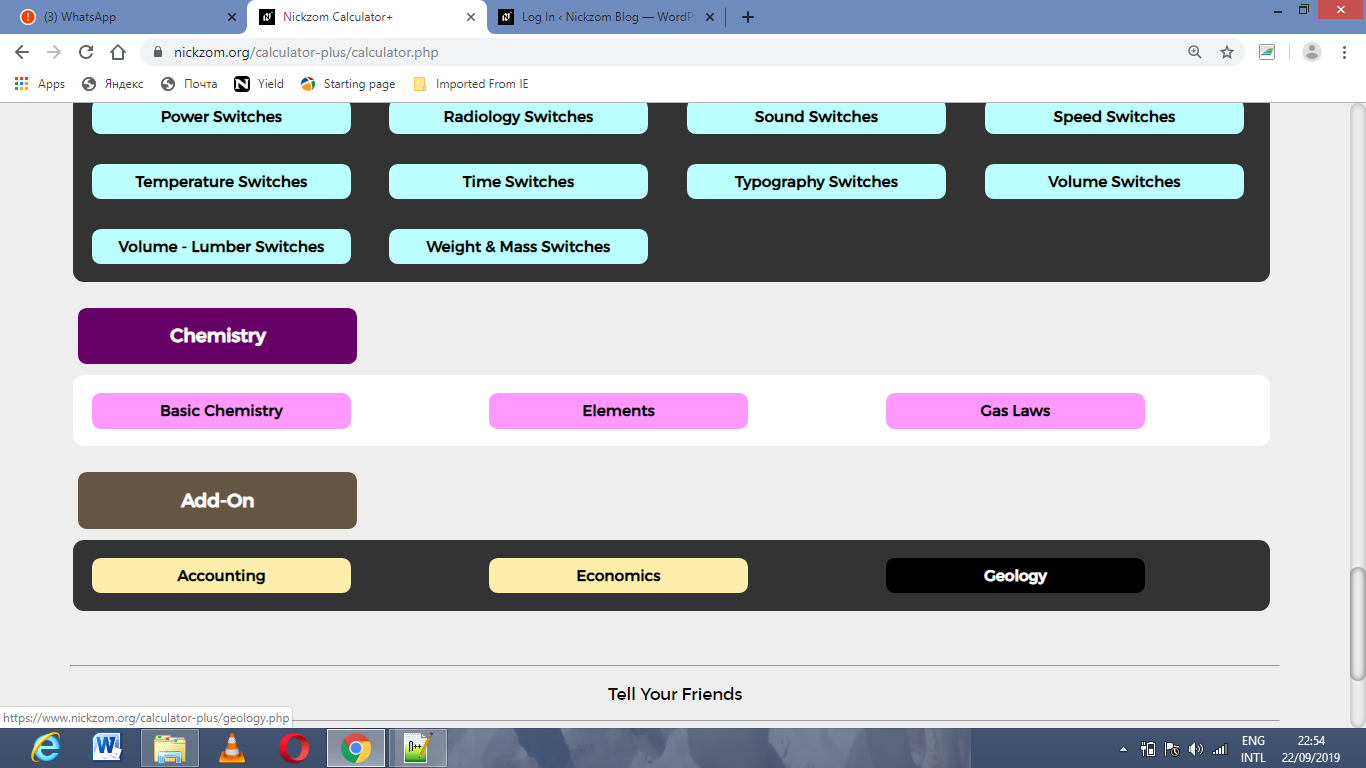Now, Click on Rock Mechanics under GeologyNow, Click on Young’s Modulus under Rock Mechanics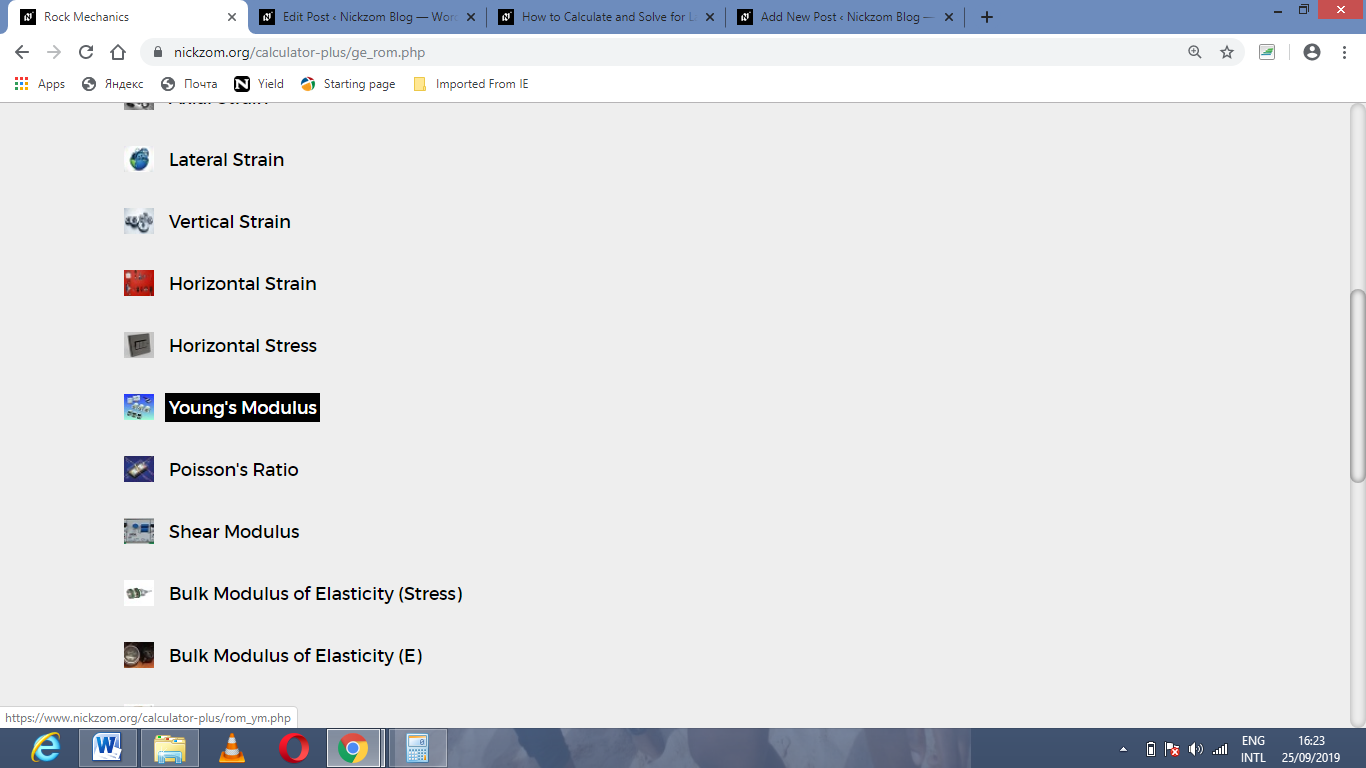The screenshot below displays the page or activity to enter your values, to get the answer for the young’s modulus according to the respective parameters which are the axial stress (σaand axial strain (εa).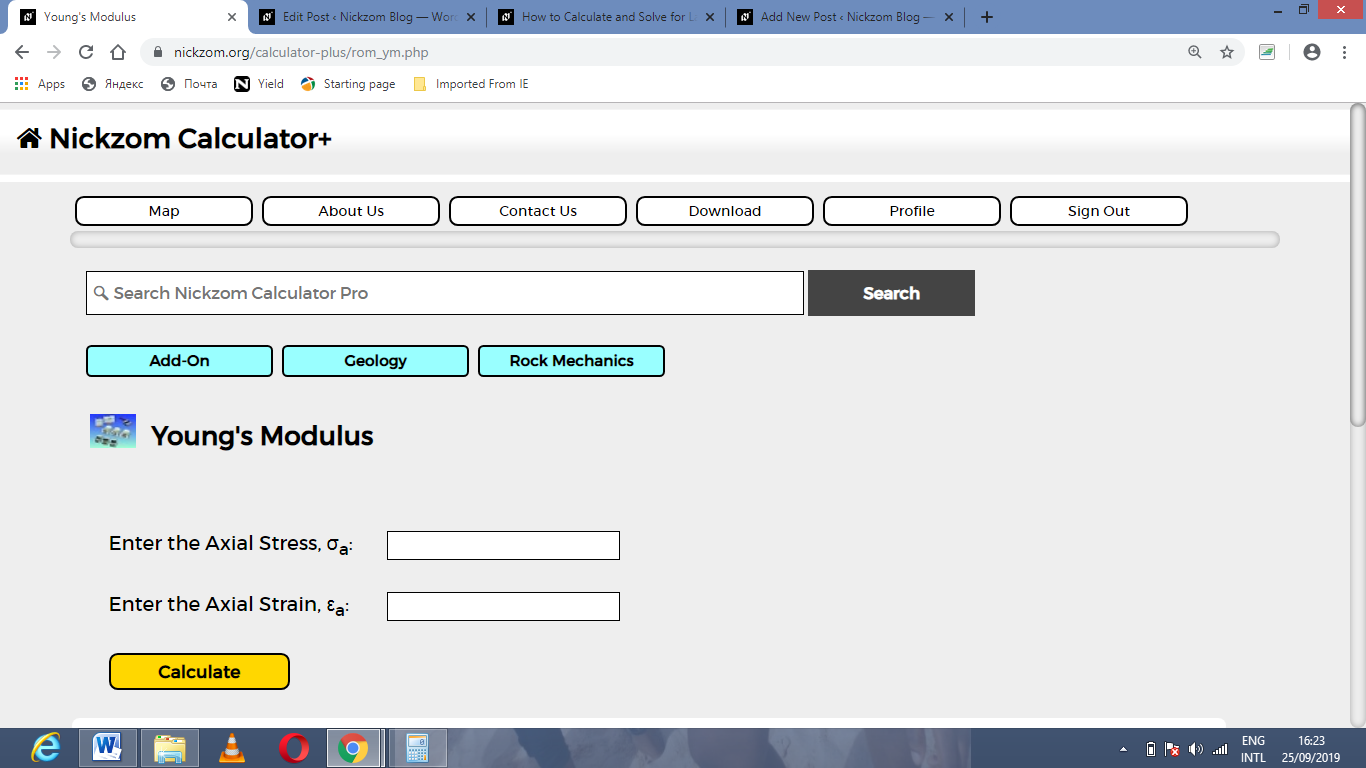Now, enter the values appropriately and accordingly for the parameters as required by the axial stress (σais 65 and axial strain (εa) is 45.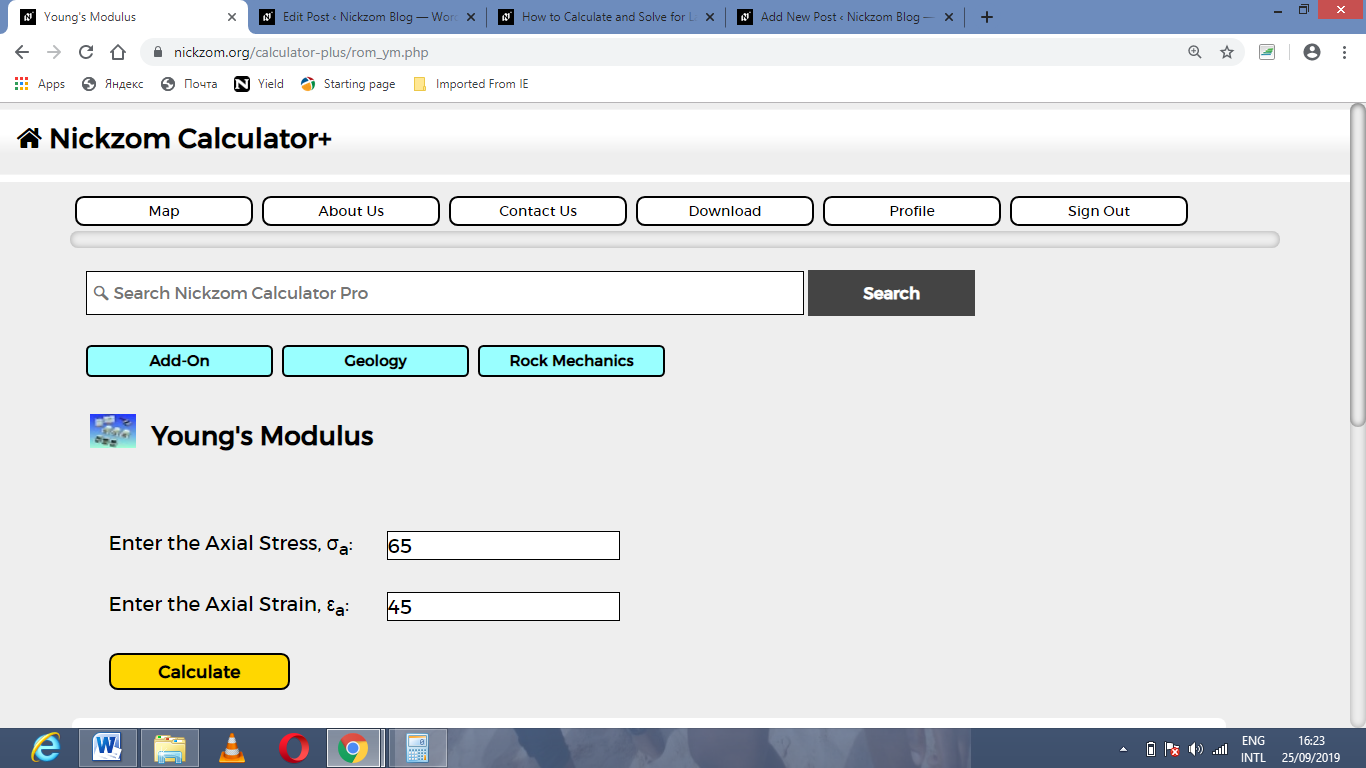Finally, Click on Calculate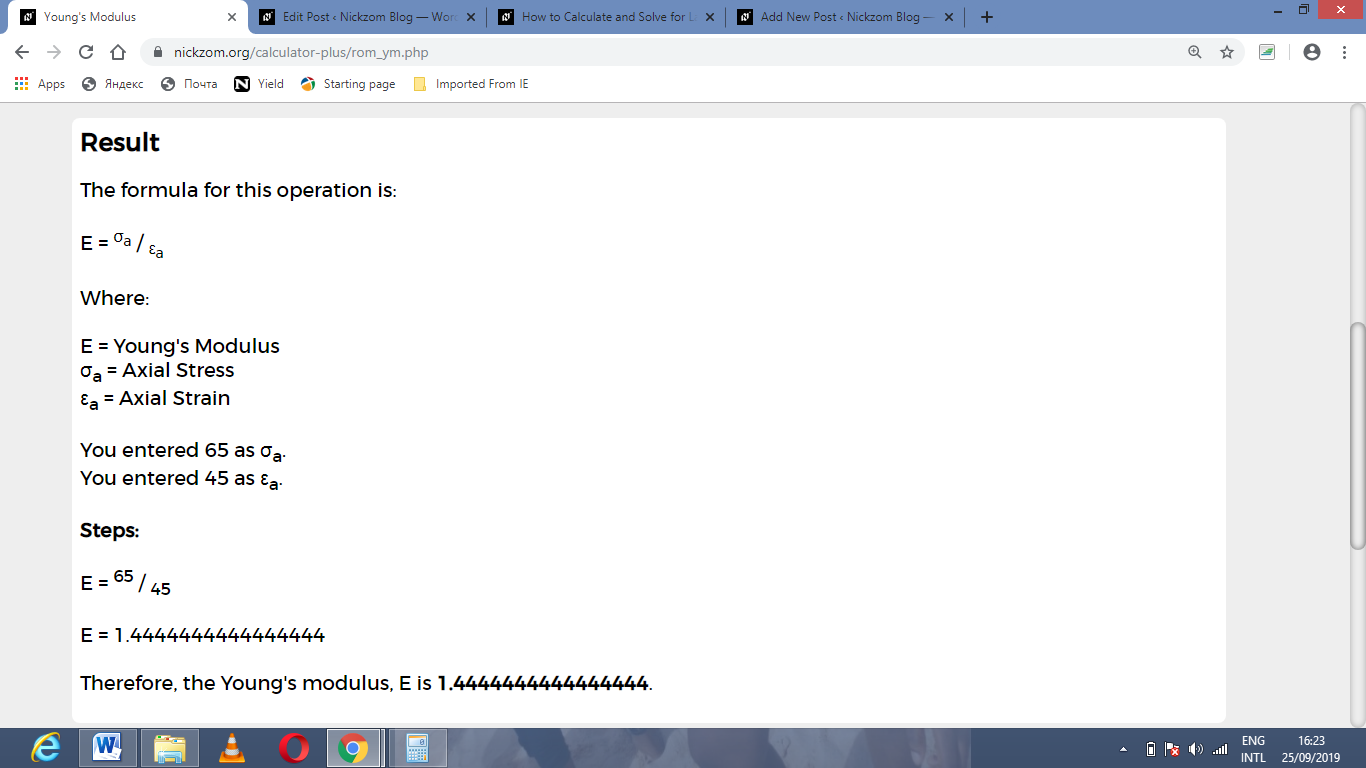As you can see from the screenshot above, Nickzom Calculator– The Calculator Encyclopedia solves for the young’s modulus and presents the formula, workings and steps too.